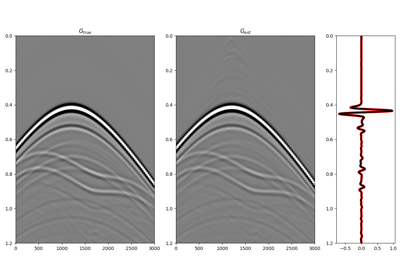# pylops.waveeqprocessing.Marchenko#

class pylops.waveeqprocessing.Marchenko(R, dt=0.004, nt=None, dr=1.0, nfmax=None, wav=None, toff=0.0, nsmooth=10, saveRt=True, prescaled=False, fftengine='numpy', dtype='float64')[source]#

Marchenko redatuming

Solve multi-dimensional Marchenko redatuming problem using scipy.sparse.linalg.lsqr iterative solver.

Parameters
Rnumpy.ndarray

Multi-dimensional reflection response in time or frequency domain of size $$[n_s \times n_r \times n_t (n_{f_\text{max}})]$$. If provided in time, R should not be of complex type. Note that the reflection response should have already been multiplied by 2.

dtfloat, optional

Sampling of time integration axis

ntfloat, optional

Number of samples in time (not required if R is in time)

drfloat, optional

nfmaxint, optional

Index of max frequency to include in deconvolution process

wavnumpy.ndarray, optional

Wavelet to apply to direct arrival when created using trav

tofffloat, optional

Time-offset to apply to traveltime

nsmoothint, optional

Number of samples of smoothing operator to apply to window

saveRtbool, optional

Save R and R.H to speed up the computation of adjoint of pylops.signalprocessing.Fredholm1 (True) or create R.H on-the-fly (False) Note that saveRt=True will be faster but double the amount of required memory

prescaledbool, optional

Apply scaling to R (False) or not (False) when performing spatial and temporal summations within the pylops.waveeqprocessing.MDC operator. In case prescaled=True, the R is assumed to have been pre-scaled by the user.

fftenginestr, optional

New in version 1.17.0.

Engine used for fft computation (numpy, scipy or fftw)

dtypebool, optional

Type of elements in input array.

Raises
TypeError

If t is not numpy.ndarray.

MDC

Multi-dimensional convolution

MDD

Multi-dimensional deconvolution

Notes

Marchenko redatuming is a method that allows to produce correct subsurface-to-surface responses given the availability of a reflection data and a macro-velocity model .

The Marchenko equations can be written in a compact matrix form  and solved by means of iterative solvers such as LSQR:

$\begin{split}\begin{bmatrix} \Theta \mathbf{R} \mathbf{f_d^+} \\ \mathbf{0} \end{bmatrix} = \mathbf{I} - \begin{bmatrix} \mathbf{0} & \Theta \mathbf{R} \\ \Theta \mathbf{R^*} & \mathbf{0} \end{bmatrix} \begin{bmatrix} \mathbf{f^-} \\ \mathbf{f_m^+} \end{bmatrix}\end{split}$

Finally the subsurface Green’s functions can be obtained applying the following operator to the retrieved focusing functions

$\begin{split}\begin{bmatrix} -\mathbf{g^-} \\ \mathbf{g^{+ *}} \end{bmatrix} = \mathbf{I} - \begin{bmatrix} \mathbf{0} & \mathbf{R} \\ \mathbf{R^*} & \mathbf{0} \end{bmatrix} \begin{bmatrix} \mathbf{f^-} \\ \mathbf{f^+} \end{bmatrix}\end{split}$

Here $$\mathbf{R}$$ is the monopole-to-particle velocity seismic response (already multiplied by 2).

1

Wapenaar, K., Thorbecke, J., Van der Neut, J., Broggini, F., Slob, E., and Snieder, R., “Marchenko imaging”, Geophysics, vol. 79, pp. WA39-WA57. 2014.

2

van der Neut, J., Vasconcelos, I., and Wapenaar, K. “On Green’s function retrieval by iterative substitution of the coupled Marchenko equations”, Geophysical Journal International, vol. 203, pp. 792-813. 2015.

Attributes
nsint

Number of samples along source axis

nrint

Number of samples along receiver axis

shapetuple

Operator shape

explicitbool

Operator contains a matrix that can be solved explicitly (True) or not (False)

Methods

 __init__(R[, dt, nt, dr, nfmax, wav, toff, ...]) apply_multiplepoints(trav[, G0, nfft, rtm, ...]) Marchenko redatuming for multiple points apply_onepoint(trav[, G0, nfft, rtm, ...]) Marchenko redatuming for one point

## Examples using pylops.waveeqprocessing.Marchenko#10. Marchenko redatuming by inversion

10. Marchenko redatuming by inversion